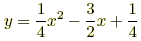index: click on a letter A B C D E F G H I J K L M N O P Q R S T U V W X Y Z A to Z index index: subject areas numbers & symbols sets, logic, proofs geometry algebra trigonometry advanced algebra & pre-calculus calculus advanced topics probability & statistics real world applications multimedia entrieswww.mathwords.com about mathwords website feedback

Directrix of a Parabola

A line perpendicular to the axis of symmetry used in the definition of a parabola. A parabola is defined as follows: For a given point, called the focus, and a given line not through the focus, called the directrix, a parabola is the locus of points such that the distance to the focus equals the distance to the directrix.Example:This is a graph of the parabolawith all its major features labeled: axis of symmetry, focus, vertex, and directrix.